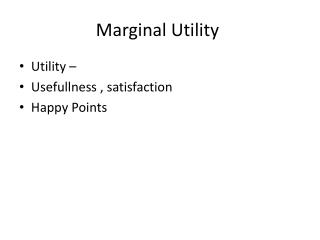DownloadDownload PresentationMarginal Utility

# Marginal Utility

Télécharger la présentation## Marginal Utility

- - - - - - - - - - - - - - - - - - - - - - - - - - - E N D - - - - - - - - - - - - - - - - - - - - - - - - - - -
##### Presentation Transcript

1. Marginal Utility • Utility – • Usefullness , satisfaction • Happy Points

2. Law of Diminishing Marginal Utility

3. Utility maximizing rule: - the consumer will maximize satisfaction when he allocates his money income so that the MU of the last dollar spent on good x is = to the MU of the last dollar spent on good y

4. MUx / Px = MU y / Py • If the equation is NOT equal (ex: MU per dollar of x = 6 , MU per dollar of y = 3) then some reallocation of the consumers expenditures (from good y to good x) will increase the consumers total utility. • As dollars are reallocated from y to x , the marginal utility per dollar of y will increase and the marginal utility per dollar of x will decrease. • *MU is diminishing with each additional good. • *for our model – consumers must use all available income

5. Marginal utility per dollar of fudge at 10 lbs. : • MU / P = 12 / \$2 = 6 • Marginal utility per dollar of coffee at 7 lbs: • MU / P = 20 / \$4 = 5 • So we conclude that as you consume more fudge, the MU will decrease and the MU per dollar will decrease, and we consume less coffee, the MU will increase and the MU per dollar will increase. • Example: • Fudge: 11 lbs MU / P = 11 / \$2 = 5.5 • Coffee: 6 lbs MU / P = 21 / \$4 = 5.25 • So the MU/P of Fudge and MU/P of Coffee get closer together

6. Simplify Know the rule: • If MU per dollar (MU / P) of good x is greater than good y; then buy more of good x and less of good y